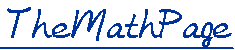Home 12.   The Circle The definition of π. The remarkable π/4. Degree measure. Standard position.The four quadrants. Coterminal angles. Radians into degrees. Degrees into radians. Coterminal angles. The multiples of π. 15.   Arc Length The definition of radian measure.  s = rθ. The area of a triangle. The ratio of the areas of similar triangles. Similar figures. All functions from one function. Complements. Cofunctions. The method of rearranging. 14.   Evaluating π The ratio of a chord to the diameter. The area of a circle algebraically. The analytic definition of the trigonometric functions. The signs in each quadrant. Quadrantal angles. The corresponding acute angle. cos (−θ) and sin (−θ).Polar coördinates. 17.   Line Values The zeros of sin θ. The period of a function. Three-place Trigonometric Table Some Theorems of Plane Geometry Home Copyright © 2022 Lawrence Spector Questions or comments? E-mail:  teacher@themathpage.com